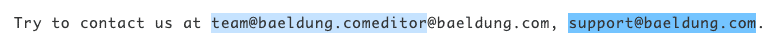## 1. Overview

Regular expressions can be used for a variety of text processing tasks, such as word-counting algorithms or validation of text inputs.

In this tutorial, we’ll take a look at how to use regular expressions to count the number of matches in some text.

## 2. Use Case

Let’s develop an algorithm capable of counting how many times a valid email appears in a string.

To detect an email address, we’ll use a simple regular expression pattern:

``([a-z0-9_.-]+)@([a-z0-9_.-]+[a-z])``

Note that this is a trivial pattern for demonstration purposes only, as the actual regex for matching valid email addresses is quite complex.

We’ll need this regular expression inside a Pattern object so we can use it:

``````Pattern EMAIL_ADDRESS_PATTERN =
Pattern.compile("([a-z0-9_.-]+)@([a-z0-9_.-]+[a-z])");``````

We’ll look at two main approaches, one of which depends on using Java 9 or later.

For our example text, we will try to find the three emails in the string:

``"You can contact me through write[email protected], [email protected], and [email protected]"``

## 3. Counting Matches for Java 8 and Older

Firstly, let’s see how to count the matches using Java 8 or older.

A simple way of counting the matches is to iterate over the find method of the Matcher class. This method attempts to find the next subsequence of the input sequence that matches the pattern:

``````Matcher countEmailMatcher = EMAIL_ADDRESS_PATTERN.matcher(TEXT_CONTAINING_EMAIL_ADDRESSES);

int count = 0;
while (countEmailMatcher.find()) {
count++;
}``````

Using this approach, we’ll find three matches, as expected:

``assertEquals(3, count);``

Note that the find method does not reset the Matcher after every match found — it resumes starting at the character after the end of the previous sequence matched, so it wouldn’t work to find overlapping email addresses.

For instance, let’s consider this example:

``````String OVERLAPPING_EMAIL_ADDRESSES = "Try to contact us at [email protected]@baeldung.com, [email protected].";

int count = 0;
while (countOverlappingEmailsMatcher.find()) {
count++;
}

assertEquals(2, count);``````

When the regex tries to find matches in the given String, first it’ll find “[email protected]” as a match. Since there’s no domain part preceding the @, the marker won’t get reset and the second “@baeldung.com” will get ignored. Moving on, it will also consider “[email protected]” as the second match:As shown above, we only have two matches in the overlapping email example.

## 4. Counting Matches for Java 9 and Later

However, if we have a newer version of Java available, we can use the results​ method of the Matcher class. This method, added in Java 9, returns a sequential stream of match results, allowing us to count the matches more easily:

``````long count = countEmailMatcher.results()
.count();

assertEquals(3, count);``````

Like we saw with find, the Matcher is not reset while processing the stream from the results method. Similarly, the results method wouldn’t work to find matches that overlap, either.

## 5. Conclusion

In this short article, we’ve learned how to count the matches of a regular expression.

Firstly, we learned how to use the find method with a while loop. Then we saw how the new Java 9 streaming method allows us to do this with less code.

As always, the code samples are available over on GitHub.

### Get started with Spring 5 and Spring Boot 2, through the Learn Spring course:

>> CHECK OUT THE COURSE